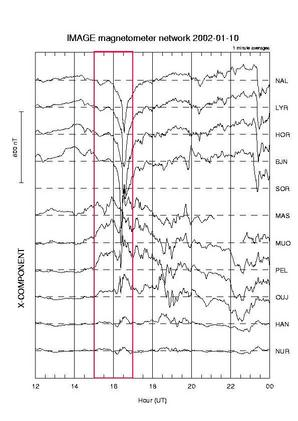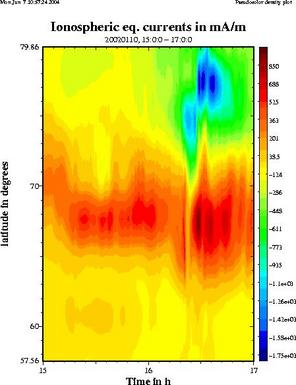## Calculate ionospheric equivalent currents (1D)Example input data:
X (northward) component data of IMAGE magnetometers on Jan 10th, 2002.
Analysis interval: 15-17 UT
(Click to get a larger version)Example output plot:
Ionospheric equivalent currents in east-west direction on Jan 10th 2002, 15-17 UT
(Click to get a larger version)

From ground magnetometers the ionospheric equivalent currents can be calculated. These are currents that flow only within the ionospheric plane (assumed at 100 km altitude) and cause the same magnetic field on the ground as the actual ionospheric current system (which may be three-dimensional). So please note: Ionospheric equivalent currents are not necessarily equal to the actual currents!

For the technique used here, we assume for simplicity that the currents are only varying in north-south direction, but not in east-west direction (electrojet-type situation). Only the east-west component of equivalent current is calculated.

The results are displayed as colour plots that show the time evolution of the equivalent currents along a north-south profile. Red colours (positive numbers) mean eastward equivalent currents, blue colours (negative numbers) mean westward currents. Four plots are displayed:

• Ionospheric equivalent currents
• Ionospheric equivalent currents, only eastward component
• Ionospheric equivalent currents, only westward component
• Integrated ionospheric equivalent current, separately for eastward and westward components

To scale the magnetic data, you need to give a baseline period, i.e. magnetically quiet period close to the event you are going to analyse. To find one, use the IMAGE IL index plots.

 More info? -> See the 1D upward continuation page under "analysis methods".

### Event selection form

NOTE: Please select the times within the same day. The script cannot handle the midnight crossing.

Event period:
Start time
 Year: Month: Day: Hour: Minute:
End time
 Year: Month: Day: Hour: Minute:
Baseline period:
Start time
 Year: Month: Day: Hour: Minute:
End time
 Year: Month: Day: Hour: Minute:
Time resolution (give number in seconds, must be integer multiple of 10)
s
(calculation will take a few seconds)